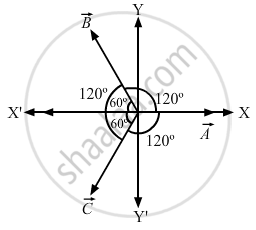Advertisement Remove all ads

# Is It Possible to Add Two Vectors of Unequal Magnitudes and Get Zero? is It Possible to Add Three Vectors of Equal Magnitudes and Get Zero? - Physics

Answer in Brief

Is it possible to add two vectors of unequal magnitudes and get zero? Is it possible to add three vectors of equal magnitudes and get zero?

Advertisement Remove all ads

#### Solution

No, it is not possible to obtain zero by adding two vectors of unequal magnitudes.
Example: Let us add two vectors  $\vec{A}$ and  $\vec{B}$ of unequal magnitudes acting in opposite directions. The resultant vector is given by

$R = \sqrt{A^2 + B^2 + 2AB\cos\theta}$

If two vectors are exactly opposite to each other, then

$\theta = 180^\circ, \cos180^\circ= - 1$

$R = \sqrt{A^2 + B^2 - 2AB}$

$\Rightarrow R = \sqrt{\left( A - B \right)^2}$

$\Rightarrow R = \left( A - B \right) \text { or } \left( B - A \right)$

From the above equation, we can say that the resultant vector is zero (R = 0) when the magnitudes of the vectors  $\vec{A}$ and $\vec{B}$ are equal (A = B) and both are acting in the opposite directions.
Yes, it is possible to add three vectors of equal magnitudes and get zero.
Lets take three vectors of equal magnitudes
$\vec{A,} \vec{B} \text { and } \vec{C}$ ,given these three vectors make an angle of $120^\circ$ with each other. Consider the figure below:Lets examine the components of the three vectors.

$A_x = A$

$A_y = 0$

$B_x = - B \cos 60^\circ$

$B_y = B \sin 60^\circ$

$C_x = - C \cos 60^\circ$

$C_y = - C \sin 60^\circ$

$\text { Here, A = B = C }$

So, along the x - axis , we have:

$A - (2A \cos 60^\circ) = 0, as \cos 60^\circ = \frac{1}{2}$

$\Rightarrow B \sin 60^\circ - C \sin 60^\circ = 0$
Hence, proved.

Is there an error in this question or solution?
Advertisement Remove all ads

#### APPEARS IN

HC Verma Class 11, 12 Concepts of Physics 1
Chapter 2 Physics and Mathematics
Short Answers | Q 2 | Page 27
Advertisement Remove all ads
Advertisement Remove all ads
Share
Notifications

View all notifications

Forgot password?